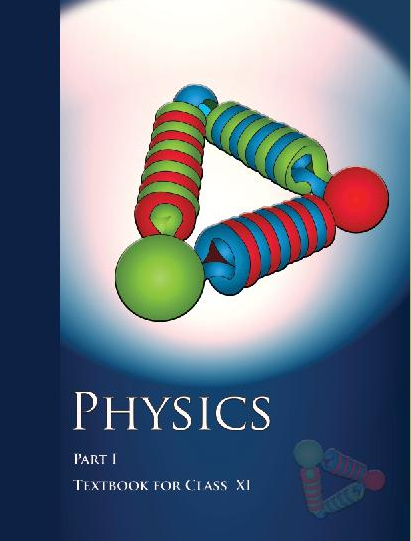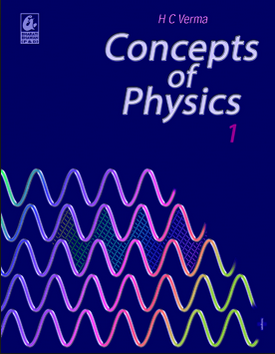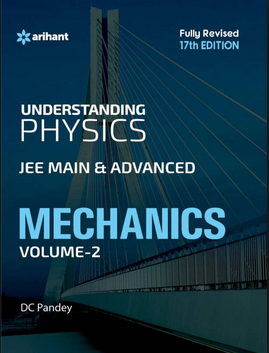# Gravitation   Share

## What is Gravitation

Have you ever wondered how you can walk on the ground freely ? or when a fruit falls from a tree or something drops from a height, it always heads for the ground? This is exactly what Sir Isaac Newton wondered and offered the world the concept of gravitation. In simple words, Gravitation is the force operating between two objects on the earth. So any thrown object will be attracted toward the earth and will fall on earth. The gravitational force has a very important role which allows us to live in this wonderful world. If gravity disappeared suddenly then we would all start to float immediately. So it is very important to study about Gravitational. And this chapter will help you to understand various laws, various properties of gravitational force along with the application of these laws and properties.

Gravitation is one of the most important chapters from mechanics while preparing for all competitive exam. Because it helps you to understand about gravitational force, field, etc which is the basis of many theories which we study under physics. Gravitation is easy to understand and a high scoring topic. You will get at least one question from this chapter. Gravitation and Electrostatics are conceptually similar to each other so when you study Electrostatics after studying gravitation, you will understand its concepts easily. The Concept of Gravitation and other chapters of mechanics are mixed in miscellaneous questions which are asked in competitive exams.

## Notes on Gravitation-

So we will discuss step by step about important topics from this chapter followed by an overview of this chapter.

Then we will understand important formulas from this chapter. Remembering these formulas will increase your speed while question-solving.

Gravitation Topics

• The universal law of gravitation.

• Acceleration due to gravity

• Kepler's laws of planetary motion.

• Gravitational potential energy; gravitational potential.

• Escape velocity. Orbital velocity of a satellite. Geostationary satellites.

Overview of Gravitation

In this chapter, you will learn about one of the fundamental force which is a gravitational force. You will also learn to calculate its magnitude with the help of formula that we get from Newton's Law of Gravitation. Now Let's try to understand the gravitational force with the help of Newton's Law of Gravitation

Prepare Online for JEE Main/NEET

Crack JEE 2021 with JEE/NEET Online Preparation Program

Newton's Law of Gravitation-

Gravitational force is an attractive force acting between two masses     and   separated by a distance r. This force is directly proportional to the  and inversely proportional toAfter studying that Gravitation force operates between any two objects on the earth, You may wonder and ask why not all bodies attract each other and stick together due to gravitational force. So answer to this question is that Gravitational Force is the weakest among all the four fundamental forces known to us till now. Let Suppose 2 similar ball of mass 1 kg are placed 1m apart then the gravitational force acting between them is simply G which is equal to    As you see this is a very negligible attractive force.

In this chapter, you will learn about various concept regarding acceleration due to gravity (g) with its definitions and suitable example.

Height, Depth, Shape of earth, Rotation of earth are the important factors which affect the value of g.

• Let's have a look at how g value changes due to the shape of the earth.So

In fact

Or we can say that Weight increases as the body are taken from equator to pole.

You will also learn about satellites and their motion in space. You will also learn to calculate their orbital velocity, their escape velocity, etc.

## Formulas for  Gravitation

1. Gravitational force-

1. Acceleration due to gravity (g)

1. Variation in 'g' with height1. Variation in 'g' with depth1. Variation in 'g' due to Rotation of earth1. Gravitational field Intensity

Where F=Gravitational force

1. Gravitational Potential

or

1. Work done against gravity

1. Escape velocity

1. Escape energy

1. Kepler's 2nd law

1. Kepler's 3rd law

or

1. Energy of satellite

## How to prepare Gravitation

• First, you should have good command over the concepts of Gravitation and you should also know how to apply them well at the time of the entrance exam or while solving questions. Please try to understand each concept from this chapter, with the help of theory, questions with solutions and video lectures on each important concept.
• For each concept practice enough problems to have a thorough understanding of the concept. Solve all the questions at home with proper concentration and try to do all calculations by yourself without seeing the solution first. Through practice, you will come to know how to approach a question• Concepts like Newton's Law of Gravitation, Acceleration due to gravity, Gravitational potential energy, Kepler’s laws of planetary motion, Escape velocity, etc are the most important concept from this chapter with respect to various competitive exam.
• Acceleration due to gravity plays a very important role to understand other concepts and solve problems easily from this chapter.
• Understand all the laws from this chapter along with their applications. With proper study, you can solve questions from this chapter with ease.

## Gravitation Tips

• Make a plan to prepare for this chapter and Stick to a Timetable.

• Study the concept first and then start solving questions. Don't go through question directly without knowing the concept.

• Solve previous year question of various exam from this chapter.

• Use smart methods to solve questions.

## Books for Gravitation

For Gravitation, chapter concepts in NCERT are enough but you will have to practice lots of questions including previous year questions and you can follow other standard books available for competitive exam preparation like Concepts of Physics (H. C. Verma) and Understanding Physics by D. C. Pandey (Arihant Publications).

## Physics Chapter wise Notes For Engineering and Medical Exams

 Chapters No. Chapters Name Chapter 1 Physics and Measurement Chapter 2 Kinematics Chapter 3 Laws of motion Chapter 4 Work Energy and Power Chapter 5 Rotational Motion Chapter 7 Properties of Solids and Liquids Chapter 8 Kinetic theory of Gases Chapter 9 Thermodynamics Chapter 10 Oscillations and Waves Chapter 11 Electrostatics Chapter 12 Current Electricity Chapter 13 Magnetic Effects of Current and Magnetism Chapter 14 Electromagnetic Induction and Alternating currents Chapter 15 Electromagnetic Waves Chapter 16 Optics Chapter 17 Dual Nature of Matter and Radiation Chapter  18 Atoms And Nuclei Chapter 19 Electronic devices Chapter 20 Communication Systems Chapter 21 Experimental skills

### Topics from Gravitation

• The law of gravitation. ( JEE Main ) (4 concepts)
• Acceleration due to gravity and its variation with altitude and depth. ( JEE Main ) (31 concepts)
• Gravitational potential energy; gravitational potential & Escape velocity. ( JEE Main ) (23 concepts)
• Kepler’s laws of planetary motion. ( JEE Main ) (4 concepts)
• Orbital velocity of a satellite. Geostationary satellites. ( JEE Main ) (20 concepts)
• Universal law of gravitation ( JEE Main ) (1 concepts)
• Acceleration due to gravity and it's variation ( JEE Main ) (6 concepts)
• Gravitational field Intensity ( JEE Main ) (6 concepts)
• Gravitational Potential ( JEE Main ) (5 concepts)
• Gravitational Potential Energy ( JEE Main ) (3 concepts)
• Kepler's laws ( JEE Main ) (1 concepts)
• Planets and satellites ( JEE Main ) (6 concepts)

### Important Books for Gravitation

•••Exams
Articles
Questions